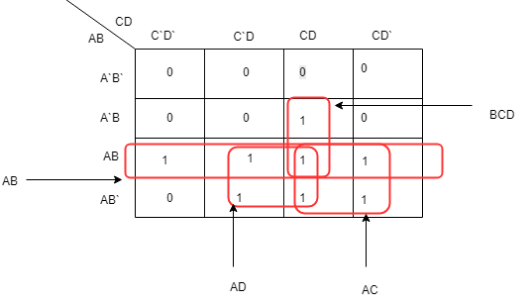# UGC NET COMPUTER SCIENCE SOLVED PAPERS 2014-16 - UGC NET Computer Science Paper 2 August 2016

>>>>>>>>UGC NET Computer Science Paper 2 August 2016

• A

(214.2)10 and (D6.8)16• B

(212.5)10 and (D6.8)16• C

(214.5)10 and (D6.8)16• D

(214.5)10 and (D6.4)16• Option : C
• Explanation : To solve this question we will apply traditional approach:
(326.4)8 = 82 * 3 + 81 * 2 + 80 * 6 . 8-1 * 4 = ( 214.5)10 is decimal representation.
For hexadecimal representation group binary sequence of (214.5) = (011010110.100)2 into group of 4.
i.e. 0 1101 0110. 1000 (0 can be padded after decimal) this is equivalent to- (D6.8)16.
So, option (C) is correct.

• A

Sign-magnitude• B

1’s complement• C

2’s complement• D

9’s complement• Option : C
• Explanation :
Sign's magnitude is only for sign convention (MSB is 1 then no is negative and if 0 then no is positive).
Main difference is that while adding numbers using 1′ s complement, we first do binary addition, then add in an end-around carry value.
But, 2′ s complement has only one value for zero, and doesn’t require carry values.
9’s complement of a decimal number is the subtraction of it’s each digits from 9.
Like 1’s complement, 9’s complement is used to subtract a number using addition.
2's complement representation is unambiguous for 0 (i.e., only positive 0), but Sign-magnitude, 1’s complement and 9’s complement are ambiguous representation for 0 (i.e., both positive and negative 0).
So, option (C) is correct.

The simplified Boolean equation for the above Karnaugh Map is

• A

AB + CD + A`B + AD• B

AB + AC + AD + BCD• C

AB + AD + BC + ACD• D

AB + AC + BC + BCD• Option : B
• Explanation :By grouping we will simply get AB + AC + AD + BCD. So, option (B) is correct.

• A

EXCLUSIVE-OR• B

EXCLUSIVE-NOR• C

NAND• D

NOR• Option : A
• Explanation :F is EXCLUSIVE-OR between X and Y. So, option (A) is correct.

 List I List II a. Controlled Inverter i. a circuit that can add 3 bits b. Full adder ii. a circuit that can add two binary numbers c. Half adder iii. a circuit that transmits a binary word or its 1's complement d. Binary adder iv. a logic circuit that adds 2 bits

Codes:

 a b c d (1) iii ii iv i (2) ii iv i iii (3) iii iv i ii (4) iii i iv ii

• A

(1)• B

(2)• C

(3)• D

(4)• Option : D
• Explanation :
Controlled Inverter is a circuit that transmits a binary word or its 1’s complement. Full adder is a circuit that can add 3 bits. Half adder is a logic circuit that adds 2 bits. Binary adder is a circuit that can add two binary numbers. So, option (D) is correct.